1

### Description

The GP2Y0A41SK0F is a distance measuring sensor unit, is very useful for detecting very close objects (4 cm to 30 cm).composed of an integrated combination of PSD (position sensitive detector) , IR-LED (infrared emitting diode) and signal processing circuit. ... This device outputs the voltage corresponding to the detection distance. So this sensor can also be used as a proximity sensor.

To test out this Sharp sensor, you'll need:

• Arduino Uno
• GP2Y0A41SK0F Sharp distance sensor

The sensor uses a 3-pin JST PH connector. These cables have a 3-pin female JST connector at one end. On the other end, the wires are unterminated, so you must strip them at the appropriate length with scissors or a wire stripper.

• If you bring up the picture of the Sharp sensor, you'll find on the right of the sensor, the position-sensitive detector. On the left, is the IR emitting diode.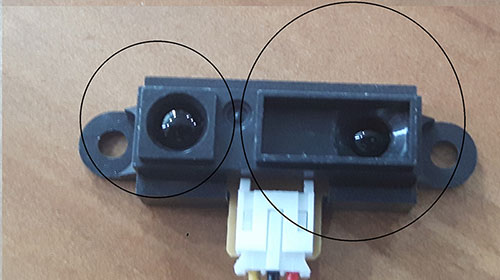How the position-sensitive detector work:

Generally, when the detector area is exposed to a light spot, the device will convert light into several outputs of electrical currents in each of the sensor's electrodes. Using the distribution of the outputs currents you can then find the light position

When you add an IR emitting diode you can make a distance sensor.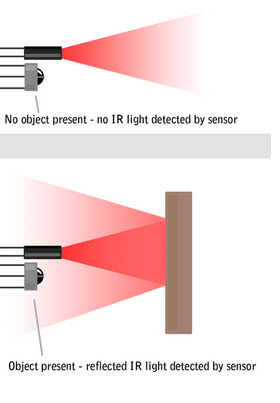The diode will produce light with a specific wavelength (IR) and the light sensor will only detect this wavelength.
When an object is close to the device, the light will bounce back on it and the intensity of this light will be measured by the sensor (the voltage in our case). The sensor will then use this data to determine the distance of the object.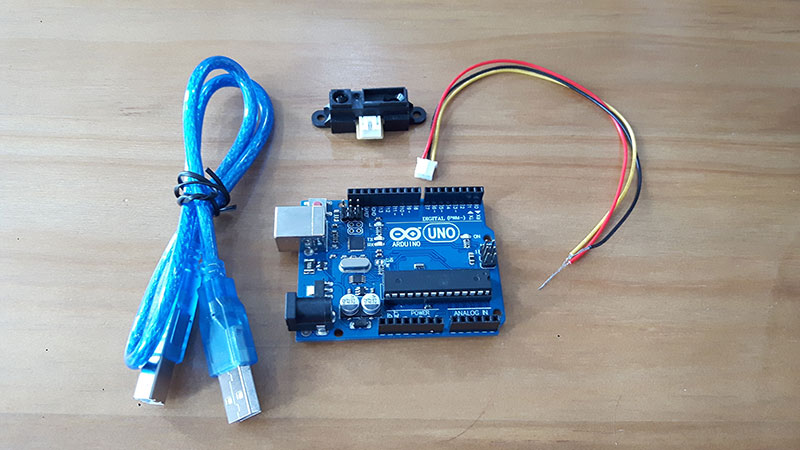#### Hook Up the Sensor and Arduino

 GP2Y0A41SK0F Sensor Arduino UNO VCC ( red wire) 5V GND (black wire) GND Signal (yellow wire) A0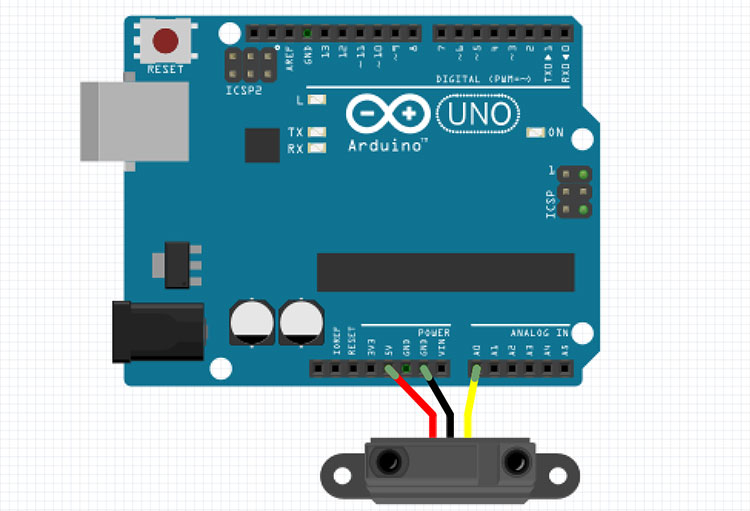```#define sensor A0 // Sharp IR GP2Y0A41SK0F (4-30cm, analog)

void setup() {
Serial.begin(9600); // start the serial port
}

void loop() {

//
float volts = analogRead(sensor)*0.0048828125;  // value from sensor * (5/1024)
Serial.println( volts);
int distance = 13*pow(volts, -1); // worked out from datasheet graph
delay(1000); // slow down serial port

if (distance <= 30){
Serial.println(distance);   // print the distance
}
}
```
• Connect your Arduino device to the computer using the USB cable
• Open your Arduino IDE (Install the IDE if you haven’t already at www.arduino.cc/en/Main/Software)
• Verify you have the correct board selected under Tools > Board
• Verify you have the correct COM Port selected under Tools > Port

Here’s### Understand the Data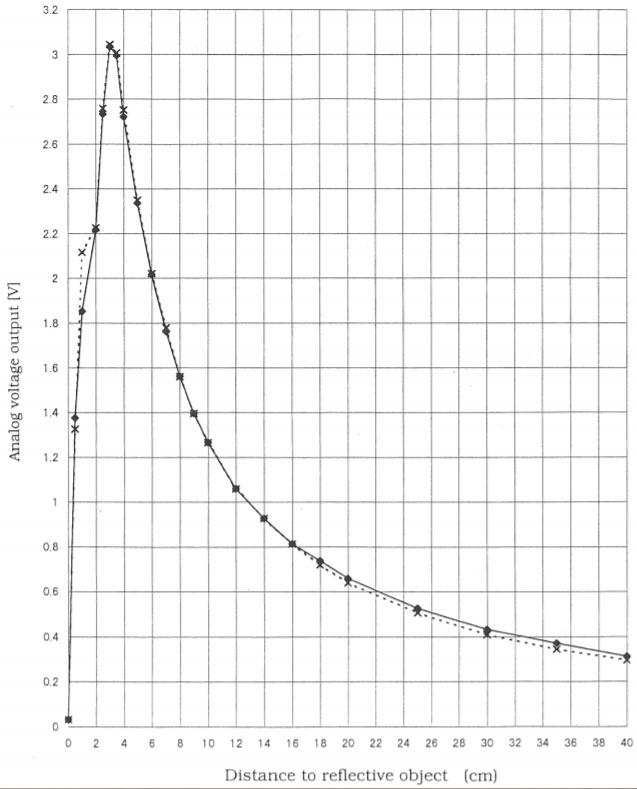Why do we multiply the value from the sensor by (5/1024)?

We convert the analogRead() value to a voltage.
The analog pins convert the value of the sensor to a byte value which is between 0 and 1023.
But we need the real analog value. To do that, we divide the voltage rating, 5V, (maximum voltage that can safely be applied to an electric device) by 1024. Then we multiply the result of this operation by the value from the sensor to get our voltage.

What is pow(volts, -1)?

In this line ‘volts’ is the base and -1 is the exponent (power to which the base is raised).
Based on the SHARP datasheet graph we can calculate the function (For distance > 3cm, it’s exponential).

The serial monitor (Tools > Serial Monitor) is a feature of the Arduino IDE that is very useful to debug your code or to control your Arduino from your computer. In our case, the distance between the sensor and the object will be printed on this monitor (9600 baud for this code).

Now that’s you’ve successfully made this sensor work, it’s time to use it for your own projects!